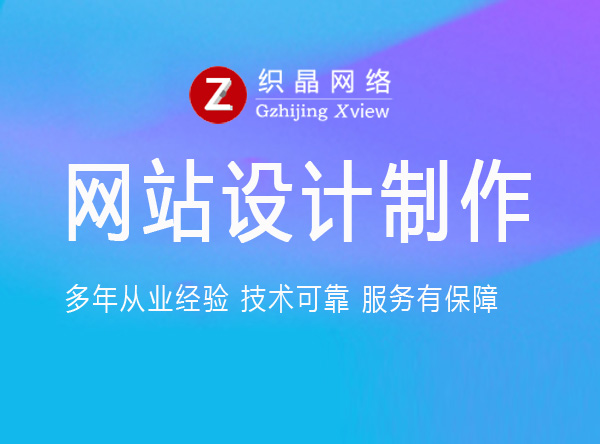• 专业的网站建设公司
• 020-39182790

#### 行业动态

##### 企业网站设计制作
###### 作者：织晶客服部   发布于：2022/9/3 16:27:35 \$(".daymd").each(function() { //console.log(\$(this).text().length); if (\$(this).text().length==17){//当日期有17个字符数 \$(this).text(\$(this).text().slice(0,8))//截取前面的8个字符 }else if (\$(this).text().length==18){ \$(this).text(\$(this).text().slice(0,9)) }else if (\$(this).text().length==19){ \$(this).text(\$(this).text().slice(0,10)) }; \$(this).text(\$(this).text().replace(/\//g,"-"));//将所有的斜杠替换成中横杠 });  点击量：  来源：织晶网络• 020-39182790
• 客服QQ
•扫一扫 加微信咨询